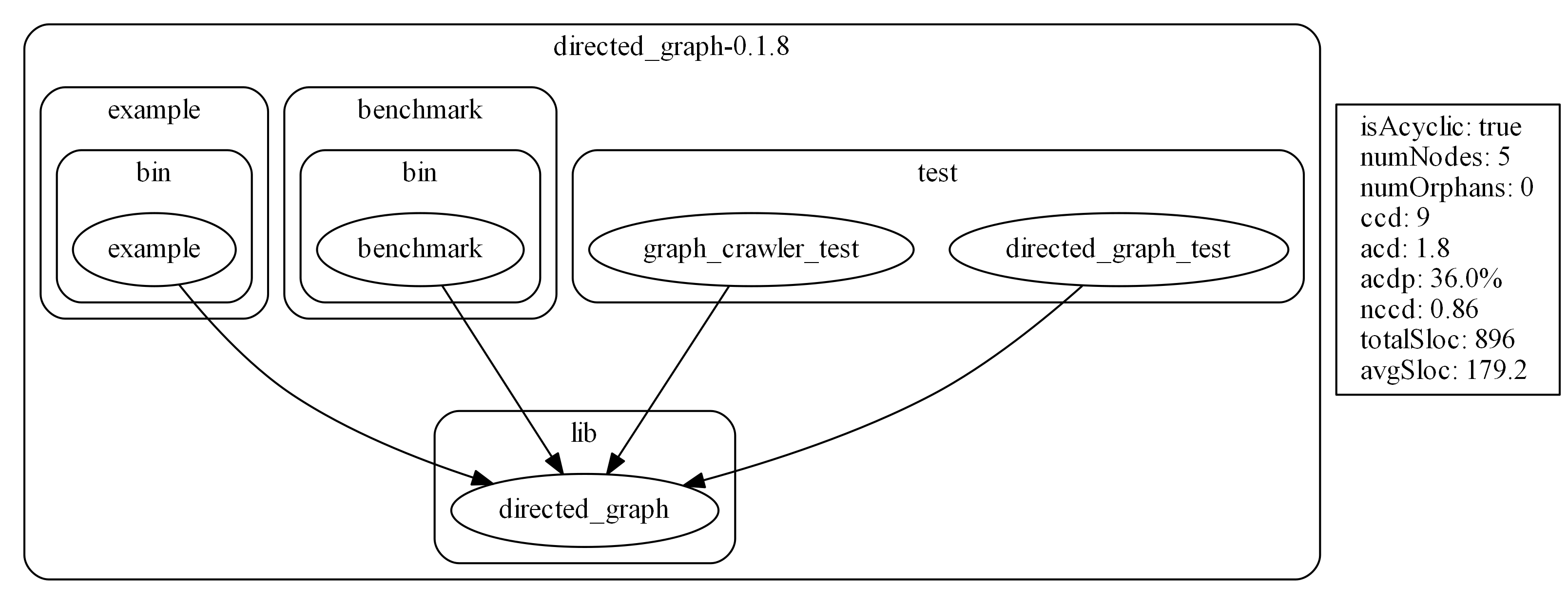`lakos` is a command line tool and library that can:

• Visualize Dart library dependencies in Graphviz dot.
• Detect dependency cycles.
• Identify orphans.
• Compute the Cumulative Component Dependency (CCD) and related metrics.

# Command Line Usage

``````Usage: lakos [options] <root-directory>

-f, --format=<FORMAT>        Output format.
[dot (default), json]

-o, --output=<FILE>          Save output to a file instead of printing it.
(defaults to "STDOUT")

--[no-]tree              Show directory structure as subgraphs.
(defaults to on)

--[no-]metrics           Compute and show global metrics.
(defaults to on)

--[no-]node-metrics      Show node metrics. Only works when --metrics is true.
--no-node-metrics is the default.

-i, --ignore=<GLOB>          Exclude files and directories with a glob pattern.
(defaults to "!**")

-l, --layout=<TB>            Graph layout direction. AKA "rankdir" in Graphviz.

[BT]               bottom to top
[LR]               left to right
[RL]               right to left
[TB] (default)     top to bottom

--[no-]cycles-allowed    Runs normally but exits with a non-zero exit code
if a dependency cycle is detected.
Only works when --metrics is true.
Useful for CI builds.
--no-cycles-allowed is the default.
``````

## Examples

Print dot graph for current directory (not very useful):

``````lakos .
``````

Pass output directly to Graphviz dot in one line (a lot more useful):

``````lakos . | dot -Tpng -Gdpi=300 -o example.png
``````

Save output to a dot file first and then generate an SVG using Graphviz dot:

``````lakos -o example.dot .
dot -Tsvg example.dot -o example.svg
``````
• Exports are drawn with a dashed edge.
• Orphan nodes are bold.
• Only `import` and `export` directives are supported; `library` and `part` are not.
• Only libraries under the root-directory are shown; Dart core and external packages are not.

## Global Metrics

Use `--metrics` to compute and show these.

isAcyclic: True if the library dependencies form a directed acyclic graph (DAG). False if the graph contains dependency cycles. True is better.

numNodes: Number of Dart libraries (nodes) in the graph.

numOrphans: Number of Dart libraries that have no imports and are imported nowhere. (inDegree and outDegree are 0.)

ccd: The Cumulative Component Dependency (CCD) is the sum of all Component Dependencies. The CCD can be interpreted as the total "coupling" of the graph. Lower is better.

acd: The Average Component Dependency (ACD). ACD = CCD / numNodes. The ACD can be interpreted as the average number of nodes that will need to change when one node changes. Lower is better.

acdp: The ACD as a percentage of numNodes. ACDP = (ACD / numNodes) * 100 = (CCD / numNodes^2) * 100. The ACDP can be interpreted as the average percentage of nodes that will need to change when one node changes. The ACDP is my original research. Lower is better.

nccd: The Normalized Cumulative Component Dependency. This is the CCD divided by a CCD of a binary tree of the same size. It's the only metric here that can compare graphs of different sizes. If the NCCD is below 1.0, the graph is "horizontal". If the NCCD is above 1.0, the graph is "vertical". If the NCCD is above 2.0, the graph probably contains cycles. Lower is better.

totalSloc: Total Source Lines of Code for all nodes.

avgSloc: The average SLOC per node. The average SLOC should arguably be kept within some Goldilocks range: not too big and not too small.

## Node Metrics

Use `--node-metrics` to show these.

cd: The Component Dependency (CD) is the number of nodes a particular node depends on directly or transitively, including itself.

inDegree: The number of nodes that depend on this node. (Number of incoming edges.)

outDegree: The number of nodes this node depends on. (Number of outgoing edges.)

instability: A node metric by Robert C. Martin related to the Stable-Dependencies Principle: Depend in the direction of stability. Instability = outDegree / (inDegree + outDegree). This yields a value from 0 to 1, where 0 means 100% stable and 1 means 100% unstable. In general, node instability should decrease in the direction of dependency. In other words, lower level nodes should be more stable and more reusable than higher level nodes.

sloc: Source Lines of Code is the number of lines of code ignoring comments and blank lines. Note: my SLOC counter function doesn't deal correctly with comments inside multi-line strings or multi-line comments in the middle of code.

## Exit Codes

Use these in your CI builds, especially DependencyCycleDetected.

1. Ok
2. InvalidOption
3. NoRootDirectorySpecified
4. BuildModelFailed
5. WriteToFileFailed
6. DependencyCycleDetected

## More Examples

Lakos run on itself, ignoring tests.

``````lakos -o dot_images/lakos.no_test.dot -i test/** .
``````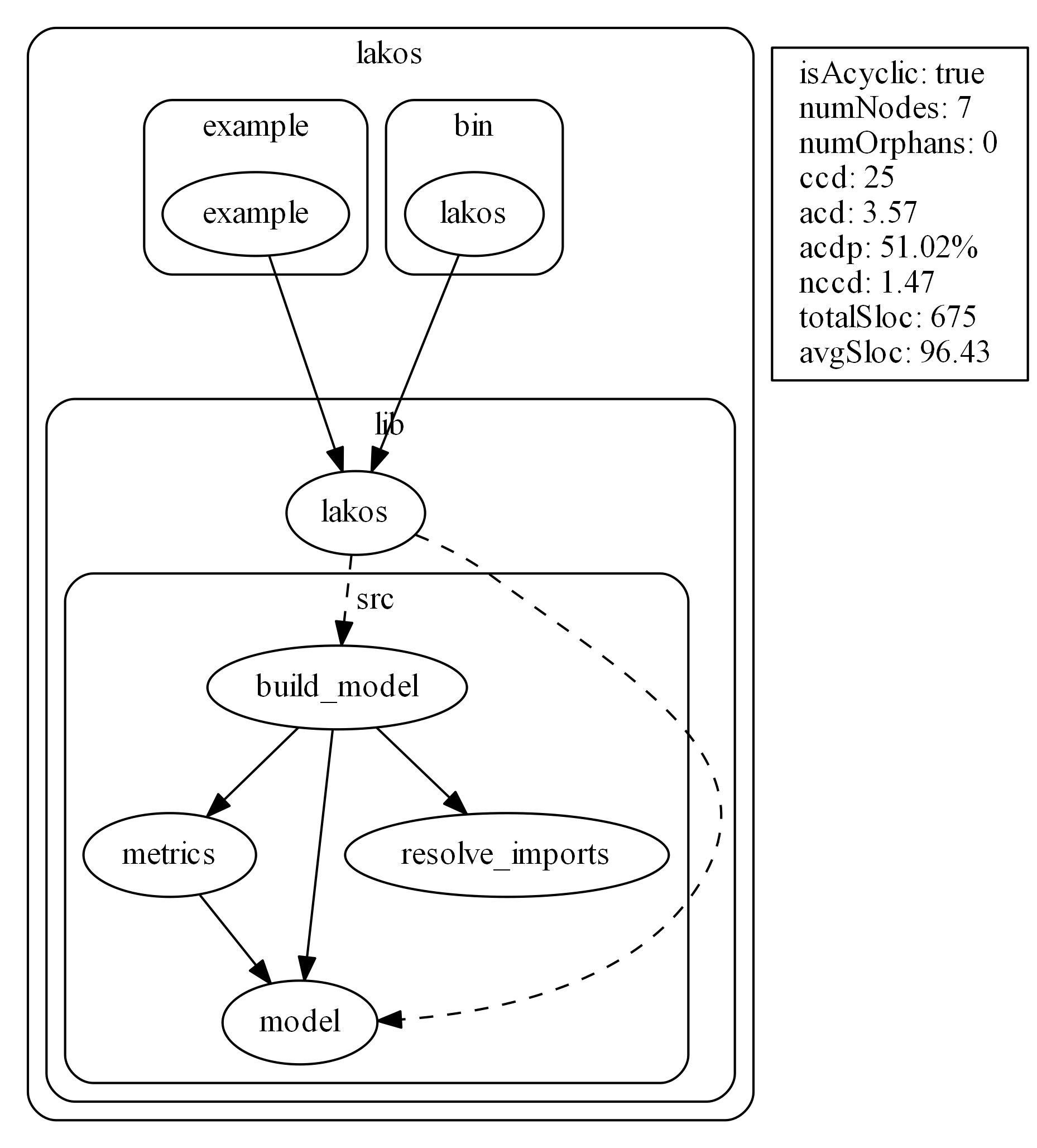No tests, show node metrics.

``````lakos -o dot_images/glob.no_test_node_metrics.dot -i test/** --node-metrics /root/.pub-cache/hosted/pub.dartlang.org/glob-1.2.0
``````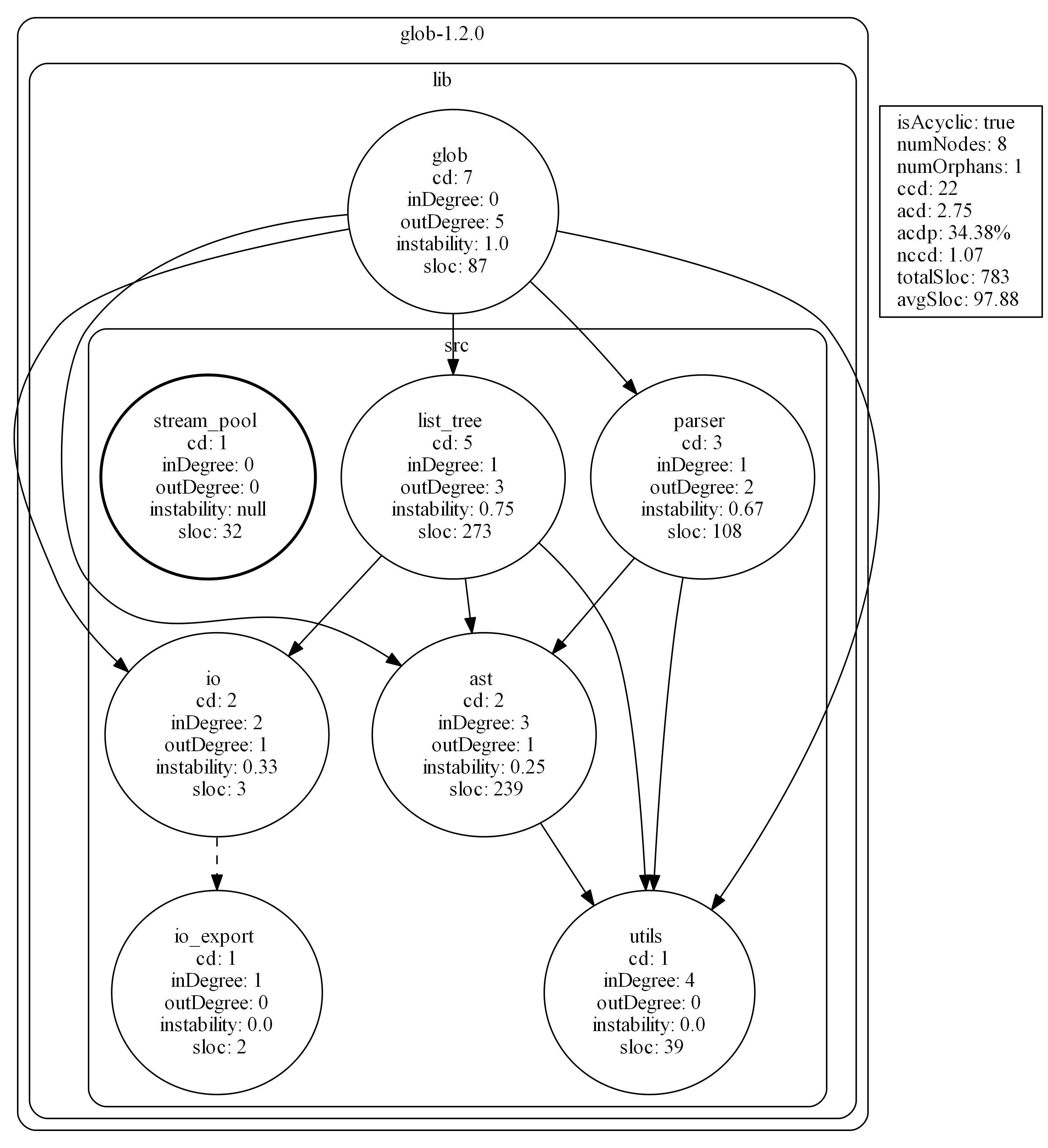No tests, no tree, no metrics.

``````lakos --no-tree --no-metrics -o dot_images/string_scanner.no_test_no_tree_no_metrics.dot -i test/** /root/.pub-cache/hosted/pub.dartlang.org/string_scanner-1.0.5
``````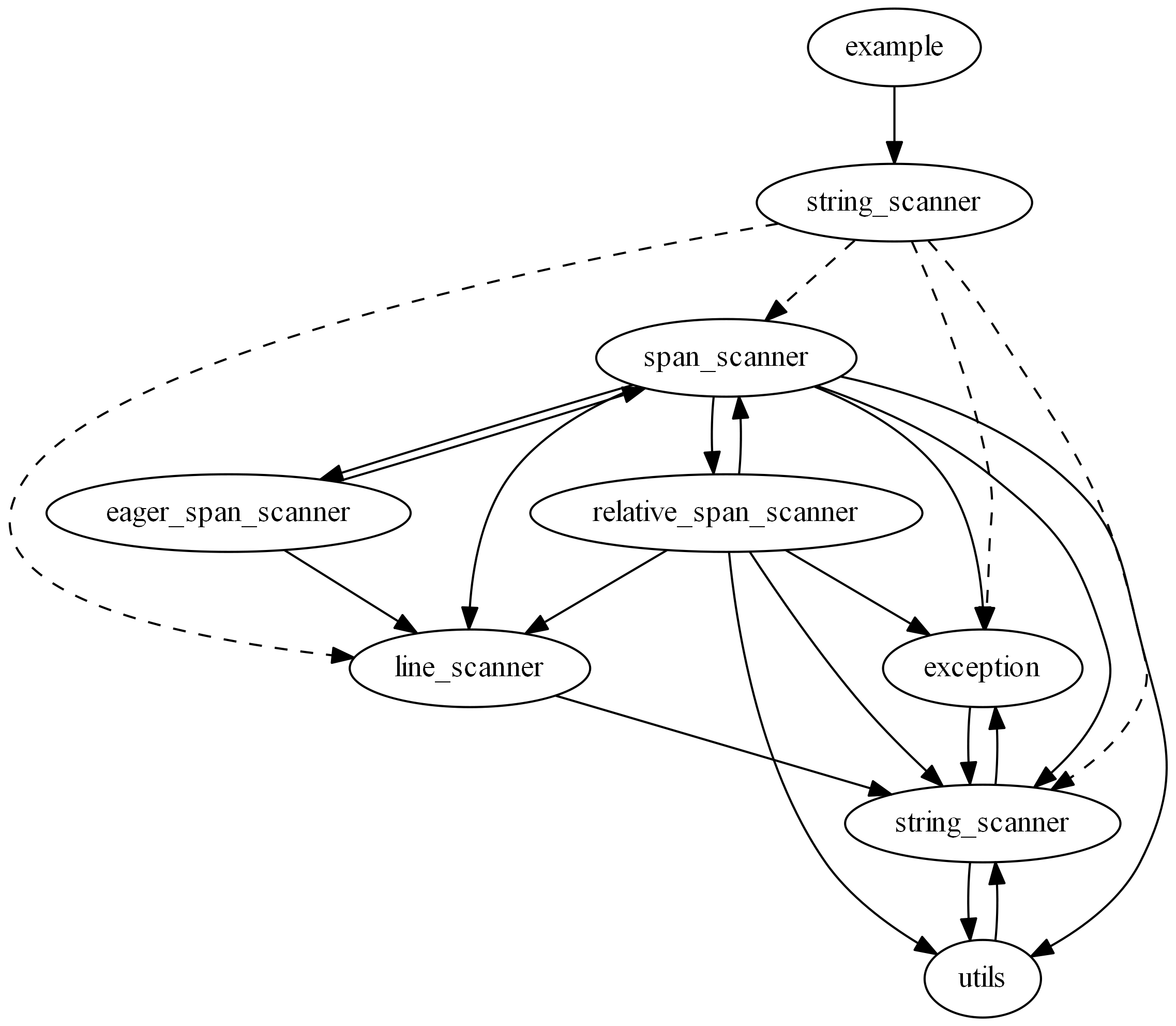No tests, no metrics, left to right layout.

``````lakos -o dot_images/test.no_test_no_metrics_lr.dot -i test/** --no-metrics -l LR /root/.pub-cache/hosted/pub.dartlang.org/test-1.15.3
``````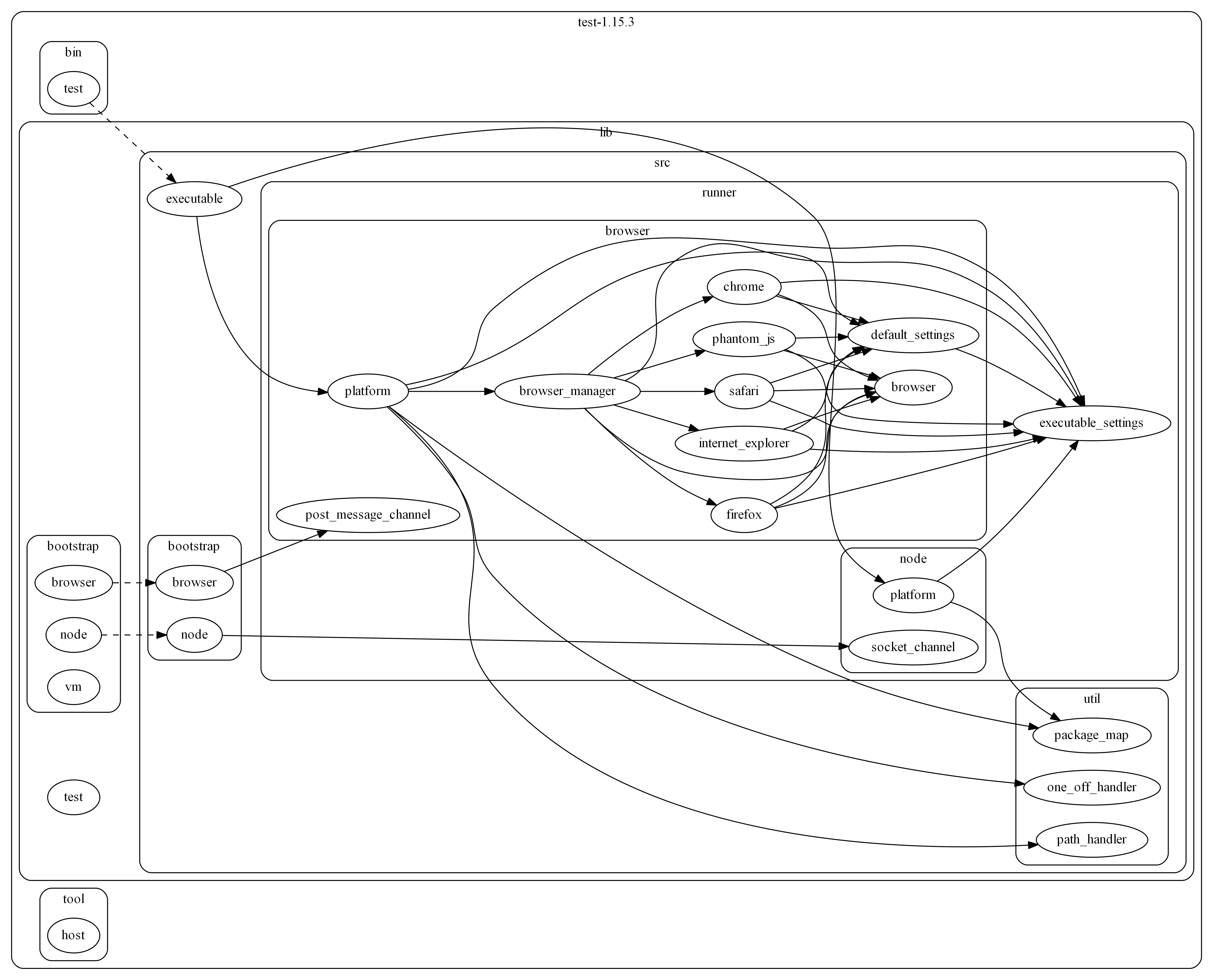A graph with circular dependencies.

``````lakos -o dot_images/path.no_test.dot -i test/** /root/.pub-cache/hosted/pub.dartlang.org/path-1.7.0
``````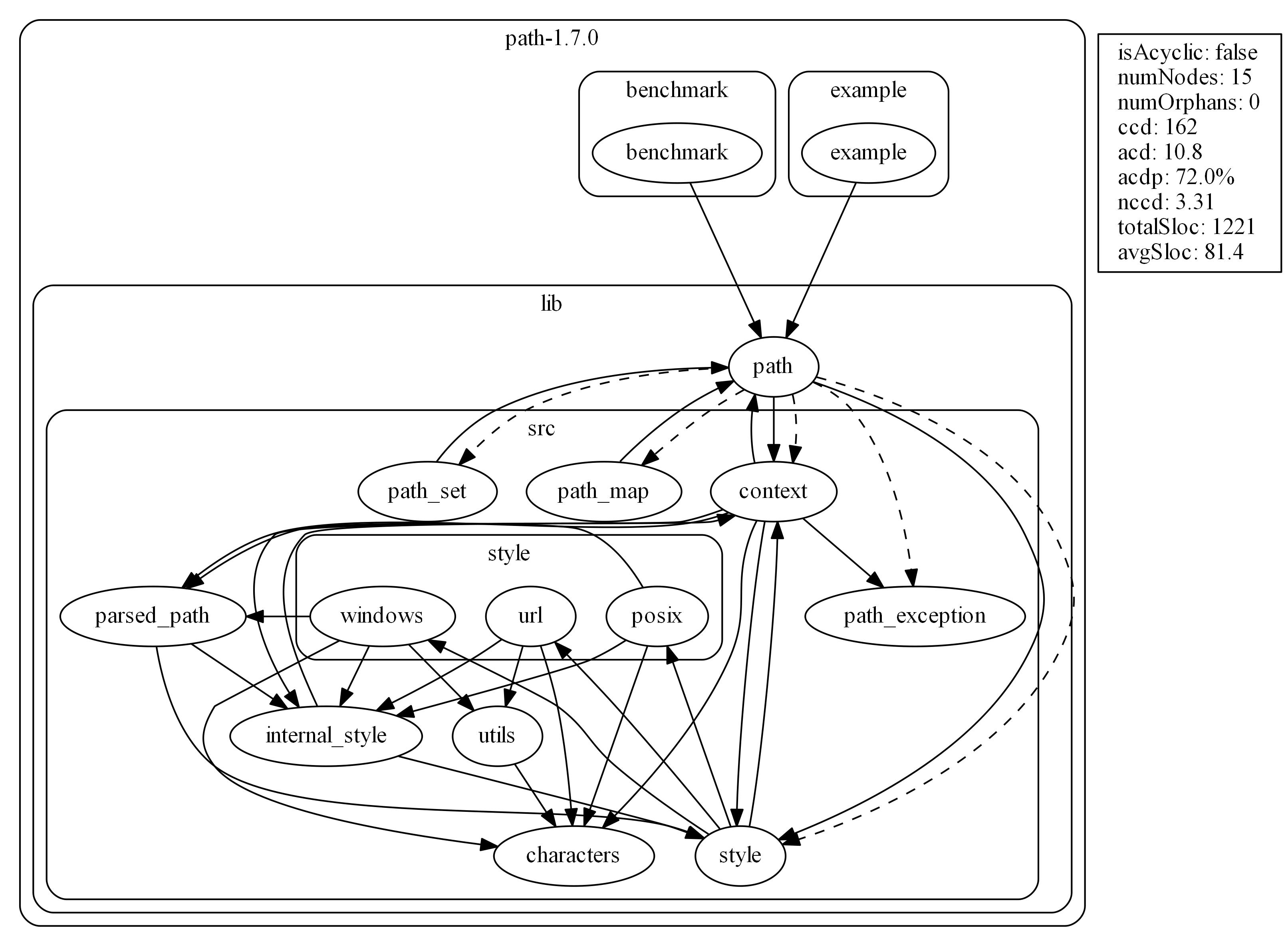Example JSON output:

``````lakos -f json -o dot_images/directed_graph.no_test.json -i test/** /root/.pub-cache/hosted/pub.dartlang.org/directed_graph-0.1.8
``````
``````{
"rootDir": "/root/.pub-cache/hosted/pub.dartlang.org/directed_graph-0.1.8",
"nodes": {
"/benchmark/bin/benchmark.dart": {
"id": "/benchmark/bin/benchmark.dart",
"label": "benchmark",
"cd": 2,
"inDegree": 0,
"outDegree": 1,
"instability": 1.0,
"sloc": 206
},
"/example/bin/example.dart": {
"id": "/example/bin/example.dart",
"label": "example",
"cd": 2,
"inDegree": 0,
"outDegree": 1,
"instability": 1.0,
"sloc": 73
},
"/lib/directed_graph.dart": {
"id": "/lib/directed_graph.dart",
"label": "directed_graph",
"cd": 1,
"inDegree": 2,
"outDegree": 0,
"instability": 0.0,
"sloc": 330
}
},
"subgraphs": [
{
"id": "/directed_graph-0.1.8",
"label": "directed_graph-0.1.8",
"nodes": [],
"subgraphs": [
{
"id": "/directed_graph-0.1.8/benchmark",
"label": "benchmark",
"nodes": [],
"subgraphs": [
{
"id": "/directed_graph-0.1.8/benchmark/bin",
"label": "bin",
"nodes": [
"/benchmark/bin/benchmark.dart"
],
"subgraphs": []
}
]
},
{
"id": "/directed_graph-0.1.8/example",
"label": "example",
"nodes": [],
"subgraphs": [
{
"id": "/directed_graph-0.1.8/example/bin",
"label": "bin",
"nodes": [
"/example/bin/example.dart"
],
"subgraphs": []
}
]
},
{
"id": "/directed_graph-0.1.8/lib",
"label": "lib",
"nodes": [
"/lib/directed_graph.dart"
],
"subgraphs": []
}
]
}
],
"edges": [
{
"from": "/benchmark/bin/benchmark.dart",
"to": "/lib/directed_graph.dart",
"directive": "import"
},
{
"from": "/example/bin/example.dart",
"to": "/lib/directed_graph.dart",
"directive": "import"
}
],
"metrics": {
"isAcyclic": true,
"numNodes": 3,
"orphans": [],
"ccd": 5,
"acd": 1.67,
"acdp": 55.56,
"nccd": 1.0,
"totalSloc": 609,
"avgSloc": 203.0
}
}
``````

# Library Usage

See example/example.dart

# Inspiration

`lakos` is named after John Lakos, author of Large-Scale C++ Software Design. This book presents:

• A graph-theoretic argument for keeping library dependencies acyclic.
• A set of "levelization techniques", such as Escalation and Demotion, to help avoid cyclic dependencies.
• A set of coupling metrics, such as the CCD, ACD, and the NCCD.
• An emphasis on physical design (files, folders) working in harmony with logical design (functions, classes).

# Similar Tools

• C/C++: cinclude2dot